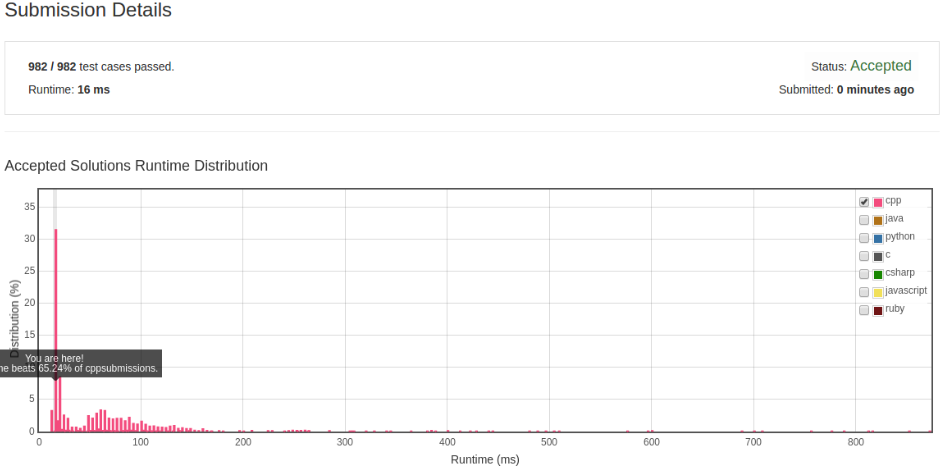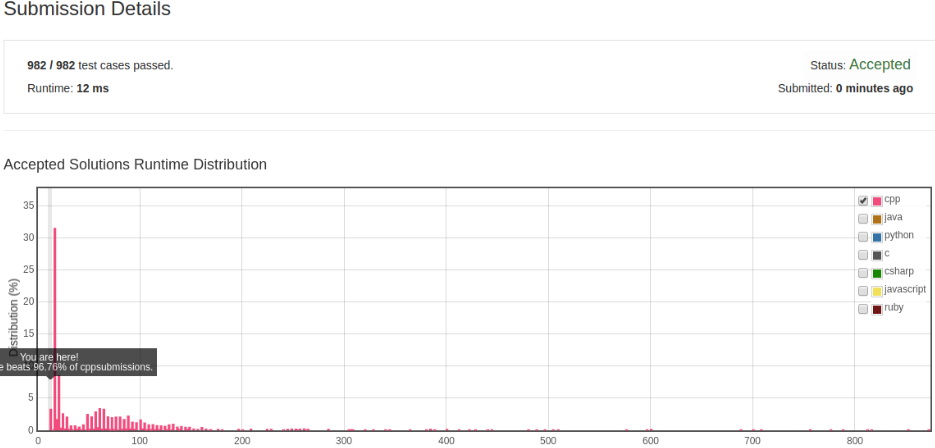# 凌云的博客

## LeetCode 算法题 3. 无重复字符的最长子串

### 题目

``````输入: "abcabcbb"

``````

``````输入: "bbbbb"

``````

``````输入: "pwwkew"

请注意，你的答案必须是 子串 的长度，"pwke" 是一个子序列，不是子串。
``````

### 思路

``````for (int j = i - count; j < curIdx; ++j) {
table[s[j]] = -1;
}

count = i - table[c];
``````

### C++ 算法实现

``````class Solution {
public:
int lengthOfLongestSubstring(string s) {
int table;
fill(table, table + 256, -1);
int curIdx = 0;
int count = 0;
int maxCount = 0;
const int size = s.size();
for (int i = 0; i != size; ++i) {
curIdx = table[s[i]];
if (-1 == curIdx) {
++count;
maxCount = max(count, maxCount);
} else {
for (int j = i - count; j < curIdx; ++j) {
table[s[j]] = -1;
}

count = i - curIdx;
}

table[s[i]] = i;
}

return maxCount;
}
};
``````### 更进一步

``````for (int j = i - count; j < curIdx; ++j) {
table[s[j]] = -1;
}

count = i - table[c];
``````

``````class Solution {
public:
int lengthOfLongestSubstring(string s) {
int table;
fill(table, table + 256, -1);
int maxCount = 0;
int noDupStart = 0;
const int size = s.size();
for (int i = 0; i != size; ++i) {
int current = s[i];
int curIdx = table[current];
if (curIdx >= noDupStart) {
maxCount = max(maxCount, i - noDupStart);
noDupStart = curIdx + 1;
}

table[current] = i;
}

return max(maxCount, int(s.size()) - noDupStart);
}
};
``````